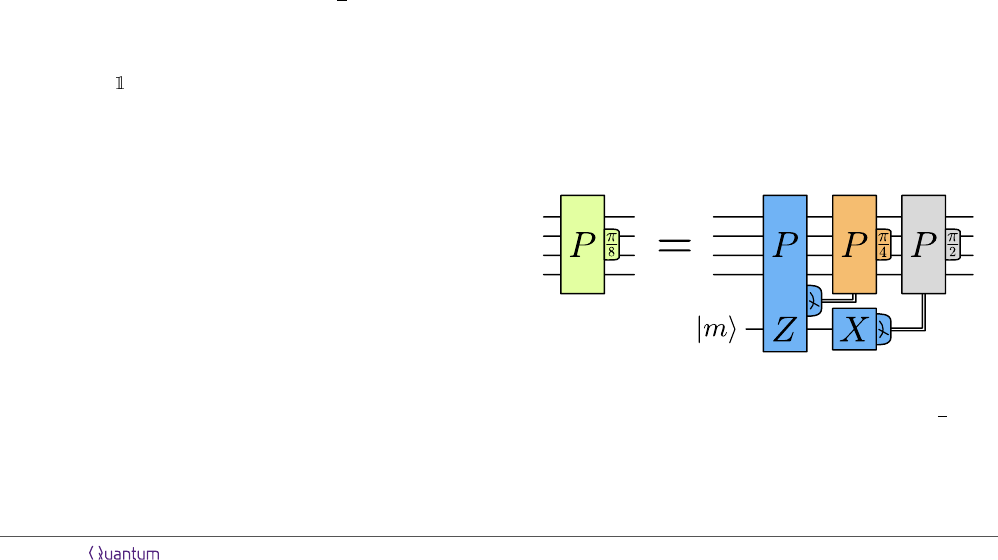Magic State Distillation: Not as Costly as You Think
Daniel Litinski @ Dahlem Center for Complex Quantum Systems, Freie Universit
¨
at Berlin, Arnimallee 14, 14195 Berlin, Germany
its ﬁrst proposal, magic state distillation is often
considered to be a very costly procedure that
dominates the resource cost of fault-tolerant
quantum computers. The goal of this work is
to demonstrate that this is not true. By writ-
ing distillation circuits in a form that separates
qubits that are capable of error detection from
those that are not, most logical qubits used for
distillation can be encoded at a very low code
distance. This signiﬁcantly reduces the space-
time cost of distillation, as well as the number
of qubits. In extreme cases, it can cost less to
distill a magic state than to perform a logical
Cliﬀord gate on full-distance logical qubits.
Quantum error correction is expected to be an es-
sential part of a large-scale quantum computer .
The most promising quantum error-correcting codes
are two-dimensional topological codes such as surface
codes [4, 5]. With these codes, the preparation of log-
ical Pauli eigenstates and the measurement of logical
Pauli product operators (e.g., via lattice surgery [6
8]) are fault-tolerant operations, which is suﬃcient for
fault-tolerant logical Cliﬀord gates. However, logical
non-Cliﬀord gates, such as T gates, cannot be executed
directly. Instead, they can be performed by preparing
a resource state that is consumed to execute a non-
Cliﬀord gate . For T gates, this resource state is a
magic state |mi = (|0i + e
/4
|1i)/
2. Such a state
can be used to perform a π/8 rotation P
π/8
= e
iP π/8
,
where P is an n-qubit Pauli product operator from the
set {X, Y, Z, }
n
. Here, X, Y and Z are Pauli op-
erators, such that Z
π/8
corresponds to a T gate. If a
magic state is available, a logical n-qubit P
π/8
gate is
performed by measuring the logical Pauli product P Z
acting on the n qubits and the magic state, see Fig. 1.
The problem with magic states is that, with surface
codes, only faulty magic states can be prepared. They
are faulty in the sense that they are initialized with
an error probability proportional to the physical error
rate p
phys
, regardless of the code distance. If these
states are used to perform logical P
π/8
rotations, one
out of every 1/p
phys
logical gates is expected to be
faulty. Since faulty gates spoil the outcome of a com-
putation, but classically intractable quantum compu-
tations with a useful computational result typically in-
volve more than 10
8
T gates , low-error magic states
are required to execute gates with a low error probabil-
ity. One possibility to generate low-error magic states is
via a magic state distillation protocol. These protocols
are short error-detecting quantum computations that
use multiple high-error magic states to generate fewer
low-error states. Many such protocols [8, 1121] have
been developed since magic state distillation was ﬁrst
proposed , gradually decreasing the cost of distilla-
tion. Even though state-of-the-art protocols are orders
of magnitude more eﬃcient than the earliest propos-
als, magic state distillation is still often described as
a costly procedure and the leading contributing factor
to the overhead of fault-tolerant quantum computing,
which is the primary motivation for research into alter-
natives to magic state distillation .
In this work, we reduce the cost of distillation by an-
other order of magnitude. On the level of circuits, none
of the distillation protocols discussed in this work are
new. Rather, the circuits are written in a way that the
number of qubits is low and the circuit depth is high.
The overhead reduction is achieved by ﬁnding surface-
code implementations of these protocols in which the
code distance of each surface-code patch is never higher
than required to achieve a speciﬁc output error prob-
ability, as was previously proposed in Ref. . This
yields protocols that not only have a low space-time
cost, but also a small qubit footprint.
The cost of distillation. How does one quantify
the cost of a distillation protocol? The space-time cost
is often quantiﬁed in units of d
3
, where d is the code
distance. However, this can be confusing, if diﬀerent
code distances are used in diﬀerent parts of the quantum
computer. Consider an n
q
-qubit quantum computation
with n
T
T gates and a quantum computer consisting of
a block of qubits used to distill magic states and a block
of qubits used to store the n
q
data qubits and consume
Figure 1: A P
π/8
rotation on n qubits can be performed by
measuring the Pauli product operator P Z acting on the n
qubits and a magic state |mi = (|0i + e
iπ/4
|1i)/
2. The
magic state is discarded via an X measurement. Measurement
outcomes of 1 of the P Z or X measurement prompt a
P
π/4
or P
π/2
correction, respectively.
Accepted in Quantum 2019-10-30, click title to verify 1
arXiv:1905.06903v3 [quant-ph] 6 Nov 2019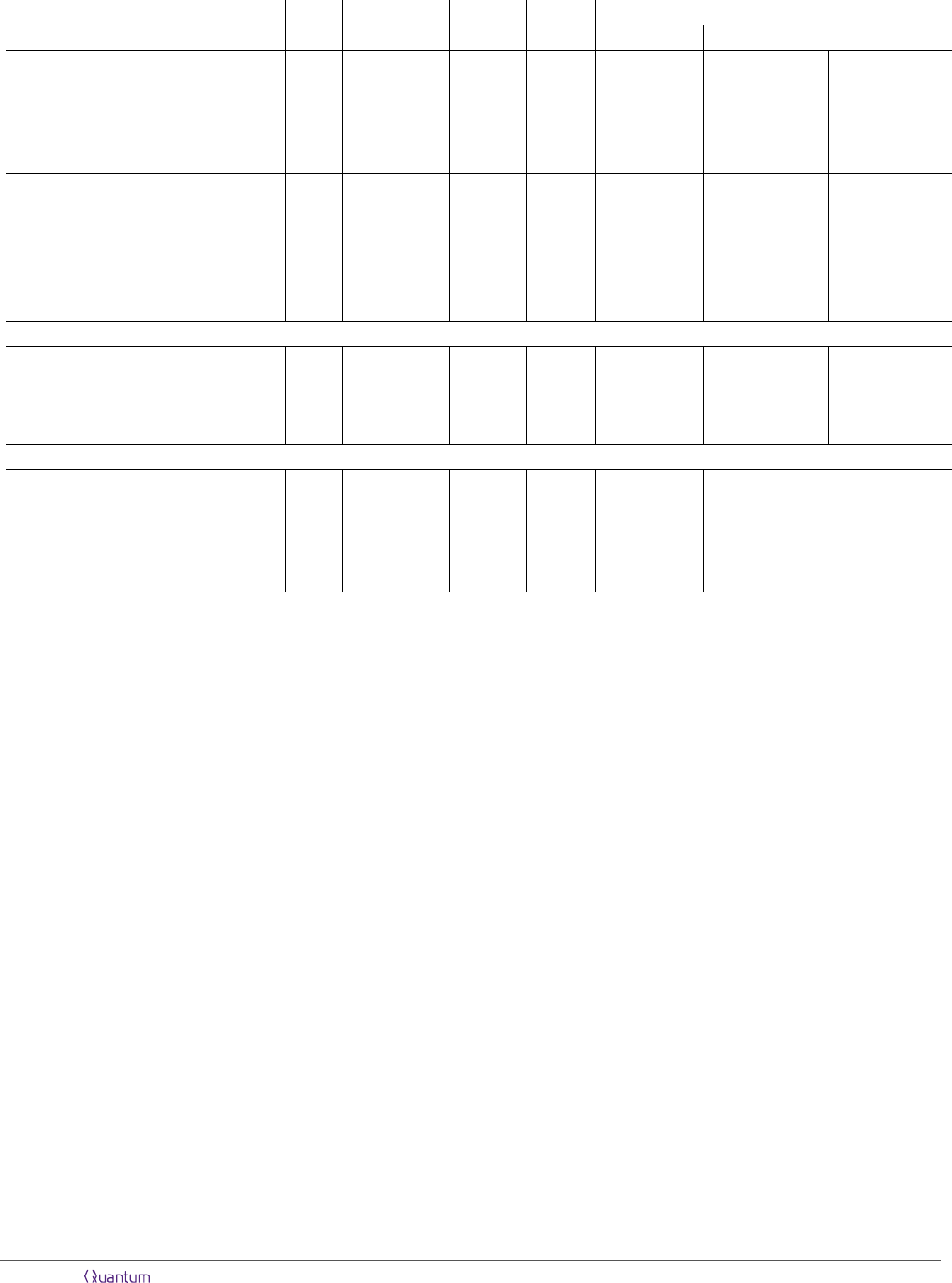Protocol p
phys
p
out
Qubits Cycles
Space-time cost per output state
Qubitcycles Full distance
(15-to-1)
7,3,3
10
4
4.4 × 10
8
810 18.1 14,600 5.49d
3
/d = 11 3.33d
3
/d = 13
(15-to-1)
9,3,3
10
4
9.3 × 10
10
1,150 18.1 20,700 4.71d
3
/d = 13 3.07d
3
/d = 15
(15-to-1)
11,5,5
10
4
1.9 × 10
11
2,070 30.0 62,000 9.19d
3
/d = 15 6.31d
3
/d = 17
(15-to-1)
4
9,3,3
× (20-to-4)
15,7,9
10
4
2.4 × 10
15
16,400 90.3 371,000 27.0d
3
/d = 19 20.0d
3
/d = 21
(15-to-1)
4
9,3,3
× (15-to-1)
25,9,9
10
4
6.3 × 10
25
18,600 67.8 1,260,000 25.9d
3
/d = 29 21.2d
3
/d = 31
(15-to-1)
17,7,7
10
3
4.5 × 10
8
4,620 42.6 197,000 6.30d
3
/d = 25 4.04d
3
/d = 29
(15-to-1)
6
13,5,5
× (20-to-4)
23,11,13
10
3
1.4 × 10
10
43,300 130 1,410,000 28.9d
3
/d = 29 19.6d
3
/d = 33
(15-to-1)
4
13,5,5
× (20-to-4)
27,13,15
10
3
2.6 × 10
11
46,800 157 1,840,000 30.9d
3
/d = 31 21.5d
3
/d = 35
(15-to-1)
6
11,5,5
× (15-to-1)
25,11,11
10
3
2.7 × 10
12
30,700 82.5 2,540,000 35.3d
3
/d = 33 25.0d
3
/d = 37
(15-to-1)
6
13,5,5
× (15-to-1)
29,11,13
10
3
3.3 × 10
14
39,100 97.5 3,810,000 37.6d
3
/d = 37 27.7d
3
/d = 41
(15-to-1)
6
17,7,7
× (15-to-1)
41,17,17
10
3
4.5 × 10
20
73,400 128 9,370,000 39.8d
3
/d = 49 31.5d
3
/d = 53
Small-footprint and synthillation protocols
(15-to-1)
9,3,3
10
4
1.5 × 10
9
762 36.2 27,600 6.27d
3
/d = 13 4.08d
3
/d = 15
(15-to-1)
9,5,5
× (15-to-1)
21,9,11
10
3
6.1 × 10
10
7,780 469 3,650,000 74.7d
3
/d = 29 50.7d
3
/d = 33
(15-to-1)
4
7,3,3
× (8-to-CCZ)
15,7,9
10
4
7.2 × 10
14
12,400 36.1 447,000 32.6d
3
/d = 19 24.1d
3
/d = 21
(15-to-1)
6
13,7,7
× (8-to-CCZ)
25,15,15
10
3
5.2 × 10
11
47,000 60.0 2,820,000 47.4d
3
/d = 31 32.9d
3
/d = 35
Historical numbers
(15-to-1) in Ref.  10
4
3.5 × 10
11
3,720 143 532,000 121d
3
(d = 13)
(15-to-1) × (8-to-2) in Ref.  10
3
2.7 × 10
11
148,000 202 14,900,000 251d
3
(d = 31)
(15-to-1) × (8-to-CCZ) in Ref.  10
3
5.3 × 10
11
134,000 171 22,800,000 473d
3
(d = 31)
(15-to-1) × (15-to-1) in Ref.  10
3
1.0 × 10
14
177,000 202 35,700,000 599d
3
(d = 31)
(15-to-1) × (15-to-1) in Ref.  10
3
3.0 × 10
15
800,000 250 200,000,000 2544d
3
(d = 34)
Table 1: Comparison of diﬀerent distillation protocols with respect to the following characteristics: physical error rate p
phys
, output
error probability per output state p
out
, space cost in qubits, time cost in surface-code cycles, and space-time cost in qubitcycles.
The last two columns report the space-time cost in (physical data qubits) × (code cycles) measured in units of the full distance
d
3
, where d is the distance required for the data qubits of a 100-qubit (left column) or 10,000-qubit (right column) computation
with at most 1/p
out
T gates. The subscripts and superscripts in the protocol description indicate the code distances and number
of level-1 distillation blocks used in the protocol, as explained in Secs. 3-6.
magic states . The distance required for the storage
of the data qubits depends on n
q
and n
T
, as it needs to
be high enough to guarantee that the probability of an
error on any of the n
q
qubits during the entire n
T
-T gate
computation is suﬃciently low. In other words, this
distance is governed by the space-time volume of the
computation n
q
·n
T
, as weight-d/2 error strings in this
space-time volume can potentially corrupt the output of
the computation. We will refer to this distance as the
full distance. The code distances used for distillation, on
the other hand, are completely irrelevant. The protocol
merely needs to produce magic states with an output
error probability that is lower than 1/n
T
, for which it
uses a certain number of qubits for a certain number of
code cycles. Since the full distance depends on n
q
, but
the space-time cost of a distillation protocol does not,
it is more meaningful to quantify the space-time cost in
terms of qubitcycles, i.e., qubits · cycles.
Results. Table 1 shows the space-time costs of the
protocols that are constructed in the following sections.
These protocols generate states with diﬀerent output
error probabilities p
out
, assuming physical circuit-level
error rates p
phys
of 10
3
and 10
4
. The more T gates
need to be executed, the lower p
out
needs to be. Each
protocol is characterized by the space cost in terms of
physical qubits (including ancilla qubits) and the time
cost in terms of code cycles, where a code cycle cor-
responds to measuring all surface-code check operators
exactly once. These numbers can be multiplied to ob-
tain the space-time cost in qubitcycles. This is a mean-
ingful ﬁgure of merit that should be minimized. It is
more meaningful than only the space cost or only the
time cost, since distillation protocols can be straight-
forwardly parallelized, using twice as many qubits to
distill states twice as fast.
Even though this is not necessarily a meaningful
quantity, we report the space-time cost in terms of the
full distance d for two diﬀerent choices of d in the last
two columns of Tab. 1. While the smallest classically
intractable quantum computations require 100 qubits,
Accepted in Quantum 2019-10-30, click title to verify 2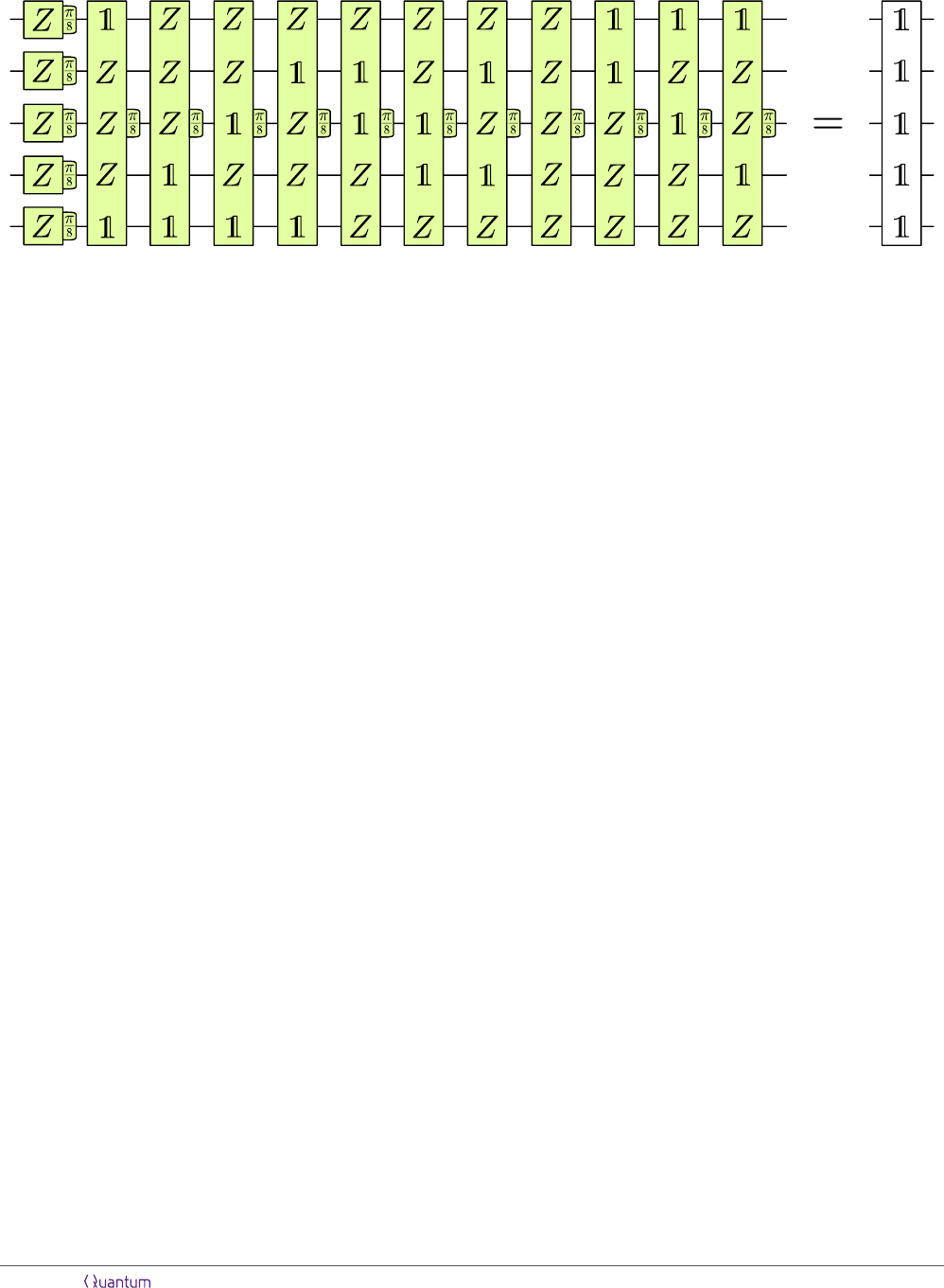Figure 2: A sequence of 16 π/8 rotations on 5 qubits that is non-trivially equivalent to the identity.
more complicated quantum algorithms use thousands of
qubits, such as factoring 2048-bit numbers using Shor’s
algorithm. The lower and higher values of d are cho-
sen such that they are suﬃcient for a 100-qubit and
10,000-qubit computation with at most 1/p
out
T gates,
respectively. The reported costs in terms of the full
distance are in terms of (physical data qubits)×(code
cycles), i.e., they do not consider physical measurement
ancillas and are therefore smaller by a factor of 2. This
is done to more easily compare the numbers to the cost
of storing a d × d surface-code patch for d code cycles,
which is 1d
3
in terms of (physical data qubits)×(code
cycles).
How to interpret the cost. Table 1 shows pro-
tocols that generate one magic state, 4 magic states,
or one |CCZi state that can be used to execute a Tof-
foli gate. For protocols that generate multiple magic
states, the space-time cost and output error are per
magic state. Our protocols feature order-of-magnitude
overhead reductions compared to the previous state of
the art for all parameter regimes. One example is the
(15-to-1)
9,3,3
protocol, where the subscripts label the
code distances used in the protocol, as explained in
Sec. 3. For p
phys
= 10
4
, it generates magic states with
p
out
= 9.3 × 10
10
, suﬃciently low for classically in-
tractable 100-qubit computations with 10
8
T gates. In
a quantum computer that can execute one T gate every
d code cycles, 231 logical qubits at d = 13 would be used
to store the 100 qubits with a low error rate , taking
into account the routing overhead. A space-time cost of
4.71d
3
for distillation implies that a footprint equivalent
to 4.71 full-distance qubits would be able to distill one
magic state every d code cycles. In this example, 2%
of the approximately 80,000 physical qubits are used for
distillation. The numbers become even more extreme
for the example of a 10,000-qubit computation with 10
8
T gates. Here, the 10,000 data qubits are stored using
20,000 logical qubits with d = 15, which means that
the space-time cost of distillation is 3.07d
3
per magic
state. For a quantum computation on more qubits or
with a lower overall error probability, distance-17 data
qubits might be required, reducing the cost to 2.11d
3
. In
this example, the cost to distill a magic state would be
lower than the space-time cost of a full-distance logical
CNOT gate, which is 3d
3
per qubit , demonstrating
that the cost of magic state distillation is not very high,
and that space-time costs that are quantiﬁed in units
of d
3
are of limited usefulness. These numbers are ad-
mittedly a bit contrived, but even in the more realistic
case of a 100-qubit computation with p
phys
= 10
3
and
p
out
10
10
, only 10% of all physical qubits are used
for distillation.
The main message is that magic state distillation is
not the dominant cost in a surface-code-based quantum
computer. Rather, the large overhead of surface codes
is due to their low encoding rate, which implies that a
large number of qubits is required to simply store all
data qubits of the computation.
Overview. In the following sections, we discuss how
the protocols in Tab. 1 are constructed. We start in
Sec. 1 by reviewing how distillation circuits work and
how their performance is quantiﬁed. Distillation pro-
tocols require faulty T gates on the level of logical
qubits, which are usually performed via state injection
and measurement. In Sec. 2, we introduce additional
protocols for faulty logical T gates based on shrinking
patches and faulty T measurements, which avoid Clif-
ford corrections and use fewer qubits and cycles. Next,
in Sec. 3, we go through the construction of the low-cost
15-to-1 protocol. In Sec. 4, we construct two-level pro-
tocols, where 15-to-1-distillation output states are fed
into a second level of 15-to-1 or 20-to-4. In Sec. 5, we
discuss synthillation protocols, i.e., the distillation of re-
source states that perform entire layers of π/8 rotations.
Speciﬁcally, we show the example of |CCZi state dis-
tillation, which can replace four T -gate magic states for
the execution of a controlled-controlled-Z gate. For the
protocols in Tab. 1, the distillation costs of CCZ states
are lower than the cost of four T -gate magic states with
a similar p
out
, indicating that synthillation can lower
Accepted in Quantum 2019-10-30, click title to verify 3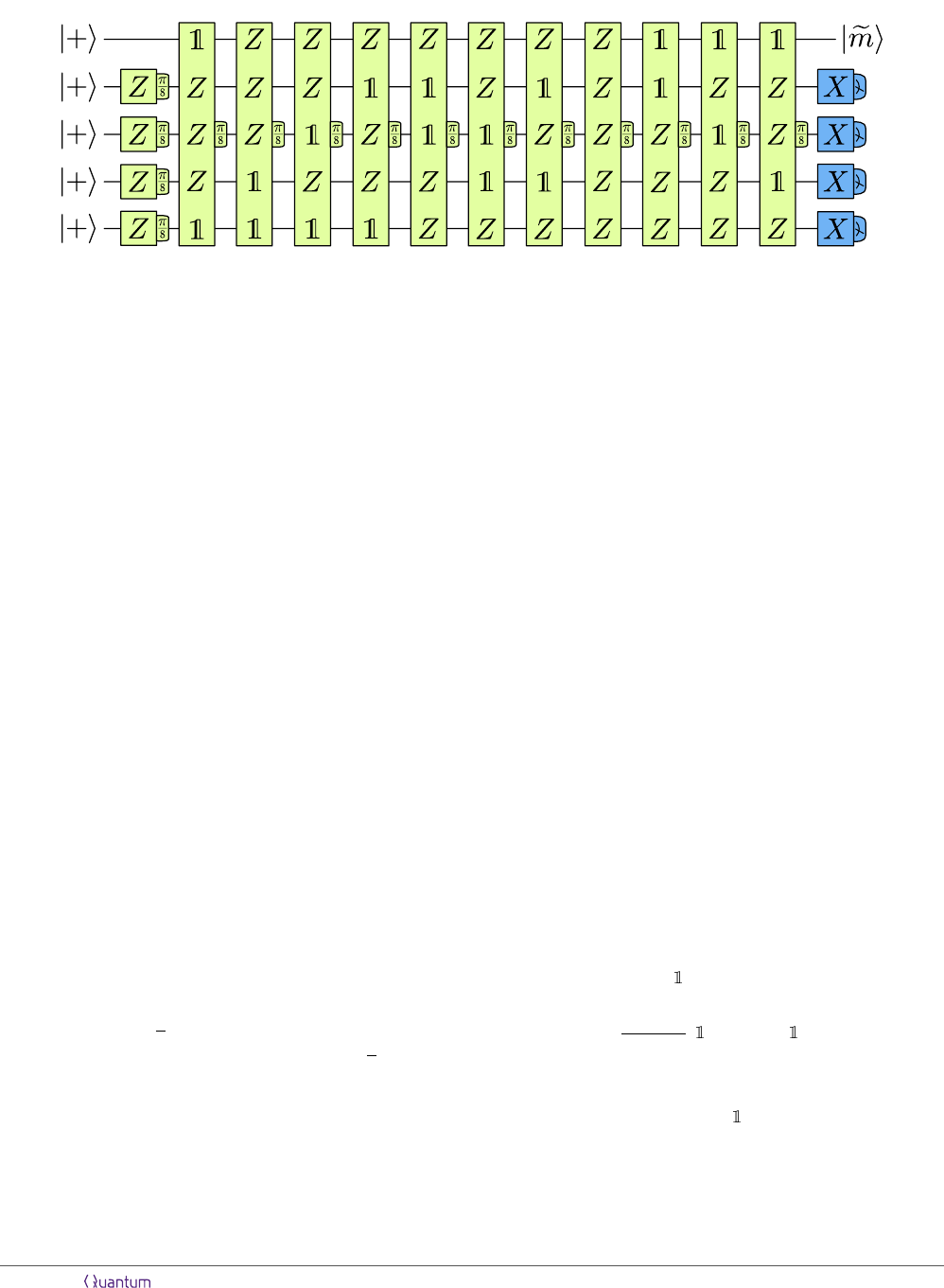5 6 7 8 9 10 11 12 13 14 151-4
Figure 3: 15-to-1 distillation circuit.
the cost compared to the distillation of T -gate magic
states. Finally, in Sec. 6, we discuss how protocols with
a higher space-time cost, but smaller qubit footprint can
be constructed. The examples shown in Tab. 1 reduce
the error rate from 10
3
or 10
4
to 10
9
, but use only
as few as 762 or 7,780 physical qubits.
1 Distillation circuits
Magic state distillation protocols can be understood in
terms of quantum error-correcting codes with transver-
sal T gates [9, 11], but it is conceptually simpler to
explain them in terms of circuits . When writing
quantum circuits as sequences of Pauli product rota-
tions P
ϕ
= e
iP ϕ
, speciﬁcally π/8 rotations P
π/8
, cer-
tain sequences are equivalent to the identity. While
some of these sequences are trivial, e.g., P
π/8
followed
by P
π/8
, there also exist non-trivial sequences. One
such sequence of 16 rotations on 5 qubits is shown in
Fig. 2. In general, such sequences are described by tri-
orthogonal matrices [11, 17]. The equivalent concept
of phase-polynomial identities is used in the context of
circuit optimization .
If we multiply the circuit in Fig. 2 by a single-qubit
rotation Z
π/8
on the ﬁrst qubit, the ﬁrst rotation will
be cancelled and the remaining circuit will consist of
15 rotations, as in Fig. 3. Since the 16-rotation cir-
cuit is equivalent to the identity, the 15-rotation cir-
cuit is equivalent to a single Z
π/8
rotation on the ﬁrst
qubit. In other words, if the initial state is |+i
5
, where
|+i = (|0i+ |1i)/
2, then the circuit prepares the state
|emi|+i
4
. Here, |emi = (|0i+e
/4
|1i)/
2 is a state
that can be used to perform π/8 rotations in the same
way as |mi, but the outcome of the P Z measurement
in Fig. 1 needs to be interpreted diﬀerently, i.e., this
state is a magic state.
Because all rotations in Fig. 3 act non-trivially on
qubits 2-5, these qubits can be used to detect errors.
If the circuit is executed without errors, qubits 2-5 are
initialized in the |+i state and returned to the |+i state,
i.e., have an outcome of +1 upon X measurement. Er-
rors are detected, if any of these measurement outcomes
are 1, in which case the protocol fails and the state is
The 15-to-1 protocol  is sometimes characterized
as having an output error probability of 35p
3
. This
assumes that every P
π/8
rotation generates a Pauli er-
ror P = P
π/2
with a probability of p. Since these are
Z-type Pauli errors, they will ﬂip all X measurement
outcomes of the qubits that they act on. Therefore,
any one faulty P
π/8
gate can be detected. Furthermore,
there is no combination of two faulty gates that can
go undetected. However, some combinations of three
faulty gates, e.g., rotations 5, 11 and 14, will cause a
Z Pauli error on the output state, but will not trigger
any ﬂipped X measurement outcomes. Since there are
35 such combinations, the probability to generate an
undetected error is 35p
3
To compute the subleading corrections to the output
error, this process can be simulated numerically. Start-
ing with the initial state ρ
init
= |+ih+|
5
, each of the
15 rotations is applied by mapping
ρ (1 p) · P
π/8
ρP
π/8
+ p · P
5π/8
ρP
5π/8
. (1)
The output state is determined by projecting into the
subspace with the correct measurement outcomes using
the projectors Π
X
= ( + X)/2, i.e.,
ρ
out
=
1
1 p
fail
( Π
4
X
)ρ( Π
4
X
) , (2)
where
p
fail
= 1 tr
( Π
4
X
)ρ
(3)
is the failure probability of the protocol. The output
error probability is computed by comparing the ideal
output state ρ
ideal
= |emihem| |+ih+|
4
to the actual
output state ρ
out
. This is done by computing the inﬁ-
Accepted in Quantum 2019-10-30, click title to verify 4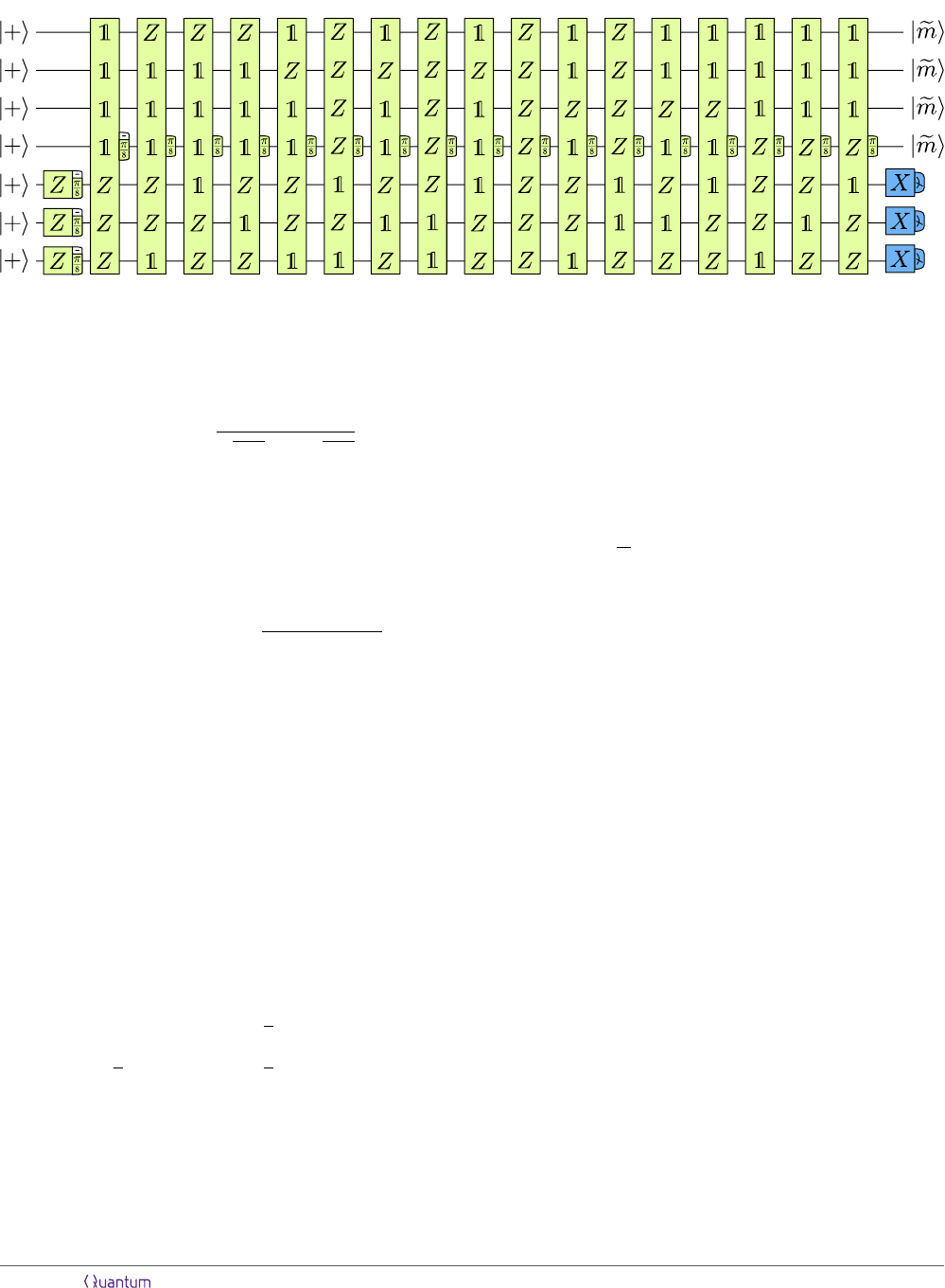4 5 6
7
8
9 11 12 13 14 15 16 17 18 19 20
1-3
10
Figure 4: 20-to-4 distillation circuit.
delity
p
out
= 1 F (ρ
ideal
, ρ
out
)
= 1 tr
q
ρ
out
ρ
ideal
ρ
out
2
= 1 tr (ρ
ideal
ρ
out
) ,
(4)
where the last equality holds, because ρ
ideal
is a pure
state. The inﬁdelity corresponds to the probability that
a faulty magic state that is used to perform a gate
in a quantum circuit will lead to an error of this cir-
cuit’s outcome . Notably, in the examples that we
consider, the trace distance tr
p
(ρ
ideal
ρ
out
)
2
/2 yields
identical or at least similar results. For the example
of p = 10
4
, the approximate output error probabil-
ity is 35p
3
= 3.5 × 10
11
, whereas the exact result is
p
out
= 3.501 × 10
11
.
Random Pauli errors. If faulty P
π/8
rotations are
performed by preparing faulty magic states and using
the circuit in Fig. 1, then the output error depends
on the error model for the preparation of faulty magic
states. In particular, if the faulty magic state is aﬀected
by a random Pauli error with probability p, i.e., by an
X, Y or Z error with probabilities p/3, respectively,
then this translates into a probability of p/3 of per-
forming either a P
π/8
, P
3π/8
or P
5π/8
of a P
π/8
rotation. In other words, after each rotation,
the state is mapped to
ρ (1 p) · P
π/8
ρP
π/8
+
p
3
· P
π/8
ρP
π/8
+
p
3
· P
3π/8
ρP
3π/8
+
p
3
· P
5π/8
ρP
5π/8
,
(5)
so there is a p/3 probability of either a P
π/4
, P
π/4
or
P
π/2
error. These ﬁrst two errors are more forgiving
than a proper P
π/2
Pauli error, since they eﬀectively
only lead to a Pauli error with 50% probability. As a
consequence, we expect each of the 35 combinations of
three faulty rotations to contribute to the output error
with (8/27)p
3
3
: Out of the 27 combina-
tions in {P
π/4
, P
π/4
, P
π/2
}
3
, there is one combination
with three P
π/2
’s, which leads to an undetected error.
There are 6 combinations with two P
π/2
error with a 50% probability, 20 combinations with one
P
π/2
leading to an error with a 25% probability, and 8
combinations with no P
π/2
’s, leading to an error with a
12.5% probability. Therefore, the output error should
be p
out
= 35 ·
8
27
p
3
10.3704p
3
deed, a numerical treatment of the full density matrix
for p = 10
4
yields p
out
= 1.03724 × 10
11
.
Coherent errors. The previous two error models
randomly applied Pauli errors with a certain probabil-
ity. One might object that, for physical qubits, this is
not necessarily a realistic error model. A more realis-
tic error model would take coherent errors into account,
such as systematic under- and over-rotation. Distilla-
tion circuits can also detect these errors, but their per-
formance is indeed worse than for incoherent errors. For
example, consider the map
ρ P
π/8+ϕ
ρP
π/8+ϕ
, (6)
which systematically over-rotates each gate by an ex-
cess angle ϕ. A gate that over-rotates by an an-
gle ϕ = arcsin(1/100) has the same gate ﬁdelity as
a gate that applies a Z error with a probability of
10
4
. However, the inﬁdelity of the output magic state
p
out
= 1.22 × 10
9
is higher by almost two orders of
magnitude compared to the incoherent case. In our
resource analysis, we will be working with incoherent
circuit-level Pauli noise, applying errors according to
Eq. (5), but with three diﬀerent probabilities for the
three diﬀerent errors. Still, we comment on how coher-
ent errors might aﬀect the output error in Sec. 4.
20-to-4 distillation. Distillation protocols can out-
put more than one magic state. If the 16-rotation circuit
in Fig. 2 is multiplied by two Z
π/8
rotations, one on the
ﬁrst and one on the second qubit, a 14-rotation circuit is
Accepted in Quantum 2019-10-30, click title to verify 5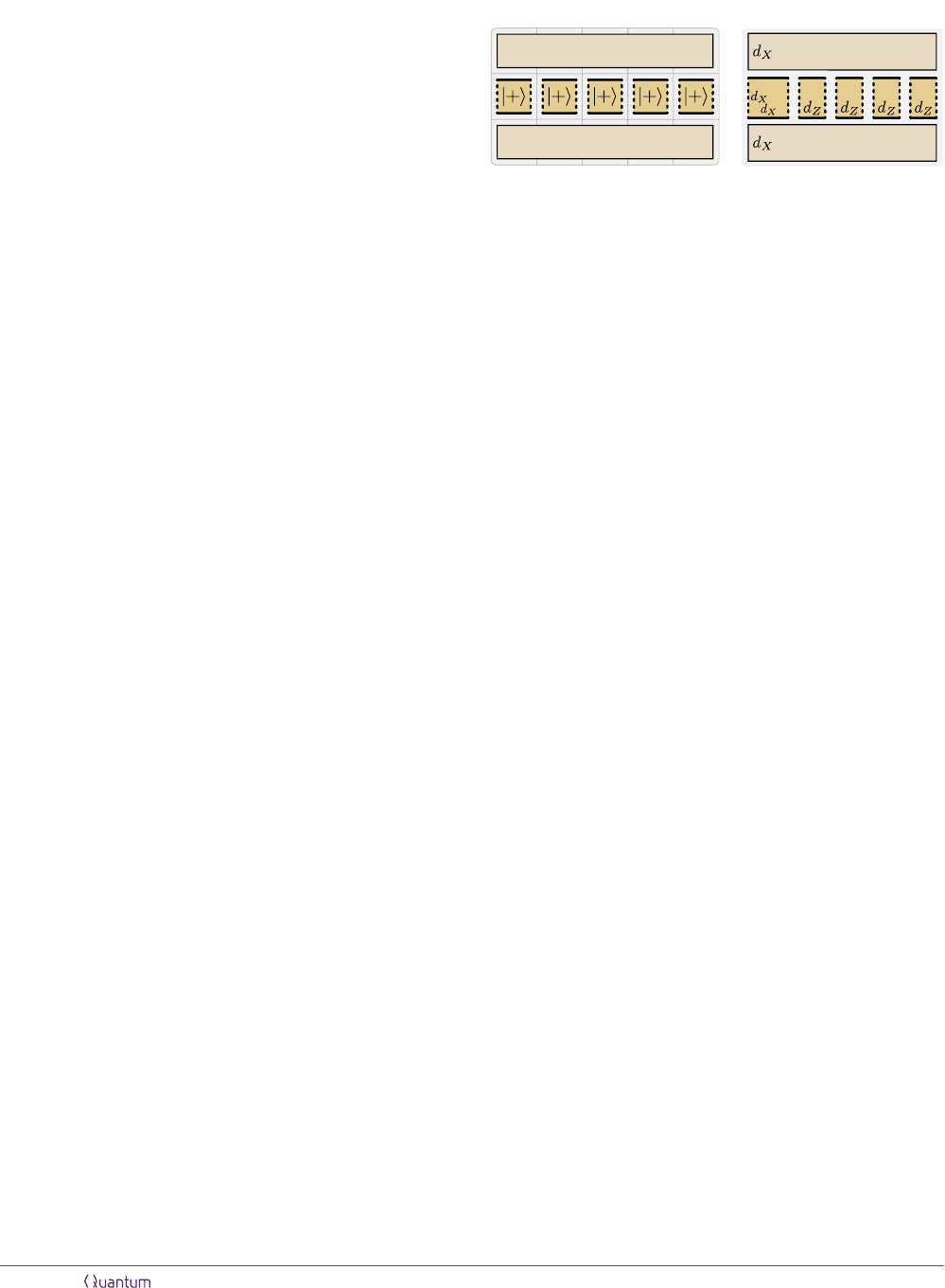obtained that outputs a |emi|emi⊗|+i
3
state, i.e., two
magic states. Similarly, using a 24-rotation circuit that
non-trivially corresponds to the identity, a 20-rotation
circuit that outputs a |emi
4
|+i
3
state can be ob-
tained. This is the 20-to-4 protocol  shown in Fig. 4.
With a Z-Pauli error model, there are 22 pairs of rota-
tions that can lead to an output error. Therefore, the
probability of an output error is 22p
2
However, since four states are produced, one should in-
terpret this as p
out
= 5.5p
2
per magic state. In other
words, the probability that the resource state |emi
4
will
cause an error in a circuit is 22p
2
, but, since this re-
source state executes four π/8 rotations, this translates
into a 5.5p
2
error probability per gate. In a numerical
simulation, the output error per state is determined via
the inﬁdelity between the projected output state and
the ideal output state |emihem|
4
|+ih+|
3
divided by
four. For p = 10
4
, this yields p
out
= 5.505 × 10
8
per
output state.
2 Faulty logical T gates
We use the notation of Ref.  to draw arrangements of
logical surface-code qubits, where patches with dashed
and solid edges represent d×d surface-code patches with
X and Z boundaries. Logical operations are performed
by measuring products of logical Pauli operators via
lattice surgery . A naive layout for the 15-to-1
protocol is shown in Fig. 5a, where the ﬁve qubits of the
15-to-1 circuit are placed next to each other with their
Z boundaries facing up and down. A 5d × d ancillary
space above and below these ﬁve qubits can be used to
measure Pauli product operators between these qubits
to perform π/8 rotations.
The code distance determines the logical error rate of
the encoded qubits, which also depends on the under-
lying error model. Here, we consider circuit-level noise,
where each physical gate, state initialization and mea-
surement outcome is aﬀected by a Pauli error with prob-
ability p
phys
. Using a minimum-weight perfect match-
ing decoder for such a noise model, the logical error rate
per code cycle  can be approximated as
p
L
(p
phys
, d) = 0.1(100p
phys
)
(d+1)/2
. (7)
Since a failure to decode X or Z syndromes correctly
leads to logical Z or X errors, respectively, we will as-
sume that logical X and Z errors each occur with a
probability of 0.5p
L
(p
phys
, d) per code cycle.
Not all errors are equally harmful in the context of
distillation protocols. Consider X and Z errors that af-
fect one of the ﬁve qubits during the 15-to-1 protocol.
Z errors aﬀecting the ﬁrst qubit (i.e., the output qubit)
are always detrimental, since they cannot be detected
ancilla
ancilla
ancilla
ancilla
(b)(a)
Figure 5: A naive arrangement (a) of logical qubits could con-
sist of ﬁve d × d patches initialized in the |+i state and two
additional 5d × d ancilla regions for Pauli product measure-
ments. The arrangement that we consider (b) consists of one
d
X
×d
X
patch, four d
Z
×d
X
patches, and two ancilla regions
with a width d
X
for Pauli product measurements.
and contribute to the overall output error of the proto-
col. The eﬀect of X errors on any of the ﬁve qubits is to
turn all previous P
π/8
rotations that acted on this qubit
into P
π/8
rotations. For instance, consider an X error
on the third qubit after rotation 7 in Fig. 3. This X er-
ror can be commuted to the beginning of the circuit and
absorbed into the initial |+i state. The commutation
turns rotations 2, 5 and 6 into π/8 rotations, since X
and Z anti-commute. As errors on multiple rotations
can lead to undetected errors, X errors should also be
avoided.
Z errors on qubits 2-5, on the other hand, are less
damaging. They are detectable, as they have the same
eﬀect as Z errors that aﬀect rotations 1-4. Therefore,
it is not necessary to encode the logical Z operators of
qubits 2-5 with the same distance as their logical X op-
erators. Instead, we encode these qubits using rectan-
gular d
X
×d
Z
patches with d
Z
d
X
. Their probability
of X errors is 0.5(d
Z
/d
X
) ·p
L
(p
phys
, d
X
) per code cycle,
since the X distance is d
X
, but the number of possible
X error strings is lower by a factor of (d
Z
/d
X
) compared
to a square patch. Correspondingly, the probability of Z
errors is 0.5(d
X
/d
Z
)·p
L
(p
phys
, d
Z
), since the Z distance
is d
Z
, but the number of Z error strings is higher by a
factor of (d
X
/d
Z
) compared to a square patch. We also
ﬁx the distance used in the ancillary region to d
X
. Fi-
nally, there is a third distance d
m
which determines the
number of code cycles used in lattice surgery. This af-
fects the error of the Pauli product measurements used
for logical gates, which can be detected by the distilla-
tion protocol.
In total, we end up with the arrangement shown in
Fig. 5b that is characterized by three code distances
d
X
, d
Z
and d
m
, where d
X
and d
Z
are spatial distances,
and d
m
is the temporal distance. Before we construct
a surface-code implementation of the 15-to-1 protocol,
we ﬁrst discuss two diﬀerent ways of performing faulty
logical π/8 rotations with surface codes: the traditional
method based on state injection, and a protocol based
Accepted in Quantum 2019-10-30, click title to verify 6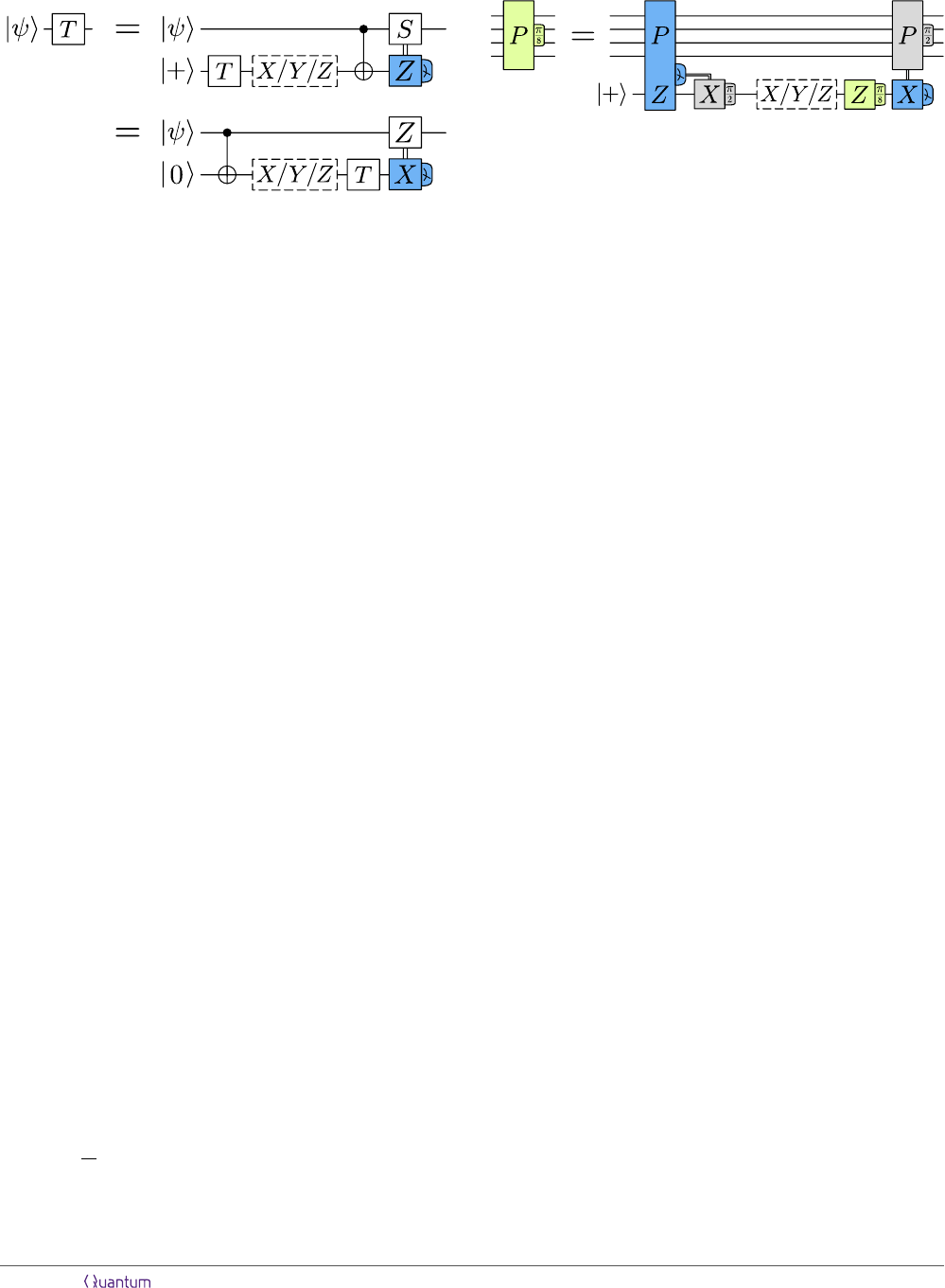(a)
(b)
Figure 6: A faulty T gate performed via state injection (a) and
a faulty T measurement (b).
on faulty T measurements.
2.1 State injection
The standard method to perform faulty T gates with
topological codes is via state injection and measure-
ment. State injection is a protocol that prepares an
arbitrary logical state |ψ
L
i from a corresponding arbi-
trary physical state |ψi. Several such state-injection
protocols exist [5, 6, 3134], but none of them are fault-
tolerant, i.e., the error probability of |ψ
L
i is always pro-
portional to p
phys
. The simplest protocol  starts with
a physical state |ψi, i.e., a 1 × 1 surface-code patch,
and then grows it into a d × 1 patch, and ﬁnally into
a d × d patch. This is not a very eﬃcient protocol,
since growing patches involves measuring stabilizers for
d code cycles. The qubit, therefore, spends many cycles
in a distance-1 state, which increases the error proba-
bility.
More sophisticated state-injection protocols use post-
selection [32, 34] to decrease the error. If the er-
ror rate of single-qubit operations is signiﬁcantly lower
than the error rate of two-qubit gates, the error due
to state injection can even be lower than p
phys
. In
circuit-level noise, a single number p
phys
characterizes
all gates. However, physical systems typically fea-
ture signiﬁcantly better single-qubit operations than
two-qubit gates. In state-of-the-art superconducting-
qubit  and ion-trap  architectures, for instance,
the ﬁdelities of single-qubit and two-qubit gates diﬀer
by up to almost two orders of magnitude. Since two-
qubit gates are typically the lowest-ﬁdelity operations,
and syndrome-readout circuits of surface codes mostly
consist of two-qubit gates, the characteristic error rate
p
phys
in circuit-level noise will be largely determined by
the error rate of two-qubit gates. If the two-qubit error
rate is p
phys
, but the single-qubit error rate is p
phys
/10,
state injection can produce magic states with an error
as low as
13
30
p
phys
 in just two code cycles. However,
there is a certain failure rate of the protocol due to post-
selection, which increases the length of the protocol.
While state injection can be used to prepare faulty
Figure 7: A faulty P
π/8
rotation corresponds to a P Z mea-
surement involving a |+i ancilla, followed by a faulty T mea-
surement of the ancilla.
magic states, it cannot be used to directly execute P
π/8
rotations. Instead, state injection is used indirectly by
preparing a faulty magic state and measuring P Z
via lattice surgery, as shown in Fig. 1. With a 50%
probability, a P
π/4
correction is required. Performing
this correction operation either requires extra time or
extra space. In any case, this Cliﬀord correction has an
eﬀect on the distillation protocol and, therefore, needs
to be performed, increasing the space-time cost of the
protocol. For this reason, we will avoid state injection,
and instead construct a protocol that executes faulty
P
π/8
rotations without the need for Cliﬀord corrections.
2.2 Faulty T measurements
When a T gate is performed on a qubit |ψi via state in-
jection, a faulty magic state is prepared, entangled with
|ψi, and measured, as shown in Fig. 6a. The faulty
preparation can be treated as a T gate applied on a
|+i state followed by a random X, Y or Z Pauli error.
The idea of faulty T measurements is to avoid the Clif-
ford correction by reversing the order of the entangling
operation and the faulty T gate, as shown in Fig. 6b.
Here, |ψi is ﬁrst entangled with a |0i qubit. Next, a
sequence of a random Pauli error, a T gate and an X
measurement is performed, which we refer to as a faulty
T measurement. Now, the correction operation in re-
sponse to the X measurement is no longer a Cliﬀord
gate, but a Pauli Z operation, which requires no addi-
tional hardware operations. X, Y and Z errors lead to
S
, S and Z errors on |ψi, respectively. Thus, a P
π/8
rotation can be performed by measuring P Z involving
an ancilla qubit initialized in the |+i state, followed by
a faulty T measurement of the ancilla qubit, as shown
in Fig. 7.
With surface codes, protocols for faulty T measure-
ments are exactly identical to protocols for state injec-
tion, except that the order of operations is reversed.
Here, we describe a simpliﬁed protocol to demonstrate
the working principle of faulty T measurements. Sim-
ilarly to the case of state injection, one can construct
signiﬁcantly more sophisticated protocols, as we discuss
in Appendix A.
One particularly simple state-injection protocol is
Accepted in Quantum 2019-10-30, click title to verify 7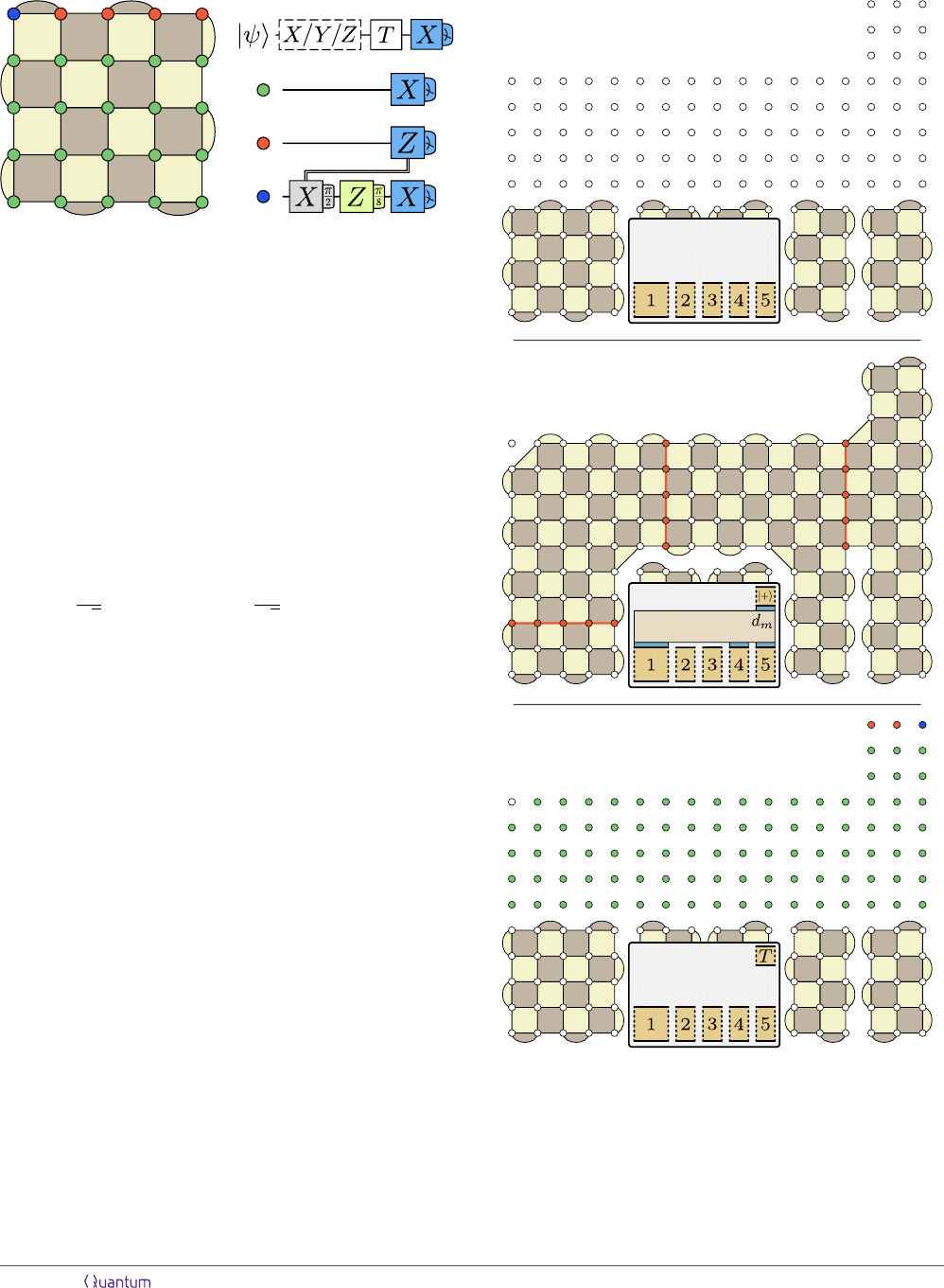Figure 8: Surface-code implementation of a faulty T measure-
ment. Bright and dark faces correspond to Z-type and X-type
stabilizers, respectively.
performed by growing a physical qubit (a 1 × 1 patch)
into a d × 1 patch and then into a d × d patch .
The corresponding faulty-T -measurement protocol can
be performed by shrinking patches. Suppose that a log-
ical qubit |ψ
L
i = α |0
L
i + β |1
L
i is encoded in a d × d
patch as in Fig. 8, with logical Z operators as strings
from left to right. This d × d patch can be shrunk to
a d × 1 patch by measuring all green qubits in the X
basis. The remaining d × 1 patch encodes the qubit in
a d-qubit XX repetition code
|ψ
L
i =
α
2
(|+i
d
+|−i
d
)+
β
2
(|+i
d
|−i
d
) , (8)
where the logical Z operator is Z
d
, and the logical X
operator corresponds to the X operator on any of the
d qubits. Next, the d × 1 patch is shrunk to a 1 × 1
patch by measuring all red qubits in the Z basis. In
fact, the red and green measurements can be performed
simultaneously. The product of all Z measurement out-
comes (and also preceding stabilizer measurements) de-
termines an X Pauli correction on the remaining qubit,
which now stores the logical information in its physical
Pauli operators. Finally, a physical T gate is applied
to the remaining qubit, before it is measured in the X
basis.
Much like state injection, faulty T measurements are
not fault-tolerant protocols, in the sense that their er-
ror rate is proportional to the physical error rate and
does not decrease with the code distance. For a Pauli
error model, these error rates can be understood as the
probabilities of the Pauli-error operations in the dashed
boxes in Fig. 6. For simplicity, we will assume that
faulty T measurements have a Pauli error rate of p
phys
,
meaning that, eﬀectively, the blue qubit is aﬀected by
an X, Y or Z error with a probability of p
phys
/3 for
each Pauli. When used to execute a P
π/8
rotation, this
implies that this gate will have a P
π/4
, P
π/4
or P
π/2
error with a probability of p
phys
/3 for each error. This
assumption is actually very inaccurate for the protocol
(1)
(2) (3)
Step 2: d
m
code cycles
Step 1: 0 code cycles
Step 3: d
m
code cycles
Figure 9: Example of a faulty T measurement to perform a
(Z
1
Z
4
Z
5
)
π/8
rotation.
shown in Fig. 8, since, for this protocol, the error scales
with the code distance d, as any single-qubit X or Y er-
ror on the red qubits translates into a logical error in the
Accepted in Quantum 2019-10-30, click title to verify 8